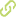# Kinematic and workspace analysis of a parallel robot used in security applications•
• Overview
•
• Identity
•
•
• View All
•

### Abstract

• This paper proposes a surveillance and defense system based on a 3SPS-1S parallel manipulator. The central constraining leg of the mechanism increases the stiffness of the system and forces the manipulator to have three pure rotation degrees of freedom. In this paper, the inverse kinematic equation is presented. The forward kinematics problem is solved using a geometrical approach, and then a unique solution to the forward kinematics is obtained using artificial neural networks and Newton-Raphson's method. A method to calculate the workspace is proposed, and then a method to maximize the workspace while maintaining the parameters of the robot as close as possible to a desired size is proposed. Finally the Jacobian matrix is obtained and analyzed in order to calculate the singularities of the manipulator. © 2013 IEEE.

### Publication date

• December 1, 2013